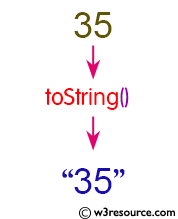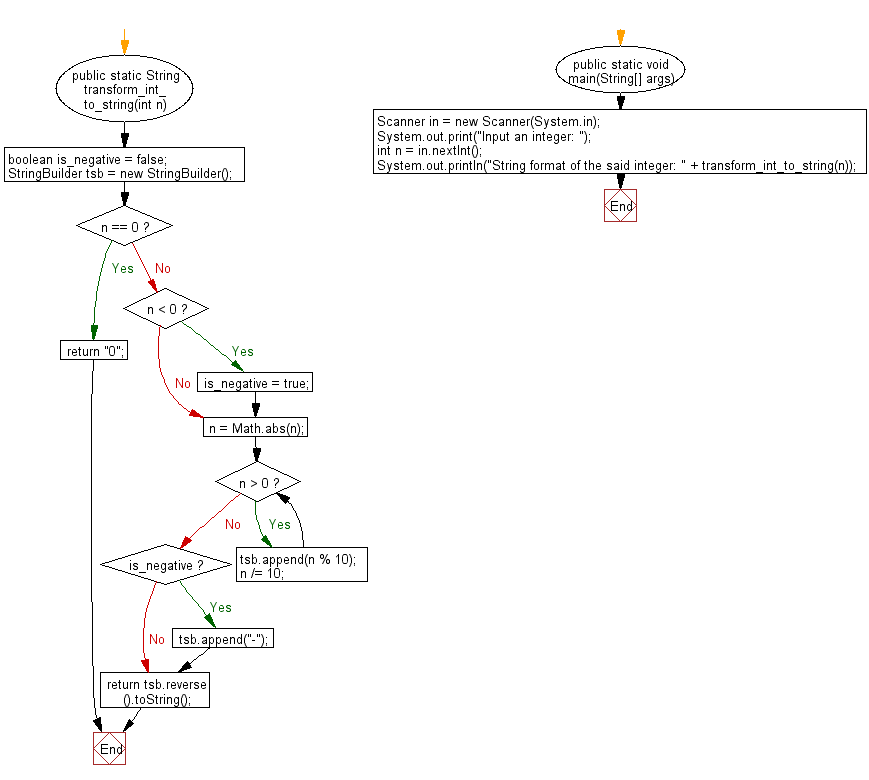﻿ Java programming exercises: Transform a given integer to String format - w3resource# Java Exercises: Transform a given integer to String format

## Java Basic: Exercise-166 with Solution

Write a Java program to transform a given integer to String format.

Pictorial Presentation:Sample Solution:

Java Code:

``````import java.util.*;
public class Solution {
public static String transform_int_to_string(int n) {
boolean is_negative = false;
StringBuilder tsb = new StringBuilder();
if (n == 0) {
return "0";
} else if (n < 0) {
is_negative = true;
}
n = Math.abs(n);
while (n > 0) {
tsb.append(n % 10);
n /= 10;
}
if (is_negative) {
tsb.append("-");
}
return tsb.reverse().toString();
}
public static void main(String[] args) {
Scanner in = new Scanner(System.in);
System.out.print("Input an integer: ");
int n = in.nextInt();
System.out.println("String format of the said integer: " + transform_int_to_string(n));
}
}
```
```

Sample Output:

```Input an integer:  35
String format of the said integer: 35
```

Flowchart:Java Code Editor:

What is the difficulty level of this exercise?

﻿

## Java: Tips of the Day

Parsing dates:

```import java.io.*;
import java.util.*;
import java.text.*;

String s = "2001/09/23 14:39";

SimpleDateFormat formatter = new SimpleDateFormat ("yyyy/MM/dd H:mm");
Date d = formatter.parse(s, new ParsePosition(0));
```